# RD Sharma Solutions For Class 7 Maths Exercise 5.4 Chapter 5 Operations On Rational Numbers

The PDF of RD Sharma Solutions for Class 7 Exercise 5.4 of Chapter 5 Operations on Rational numbers are given below. Students can access and download the PDF for free. The solutions to this exercise come with detailed explanations structured by BYJU’S expert teachers that further makes learning and understanding of concepts an easy task. This exercise includes fifteen questions all are based on the division of rational numbers. If x and y are two rational numbers such that y is not equal to zero, then the result of dividing x by y is the rational number obtained on multiplying x by reciprocal of y defines division. The division includes dividend, quotient and divisor. To know more about these concepts, we suggest you to go through the PDF of RD Sharma Solutions for Class 7 containing solutions.

## Download the PDF of RD Sharma Solutions For Class 7 Maths Chapter 5 – Operations On Rational Numbers Exercise 5.4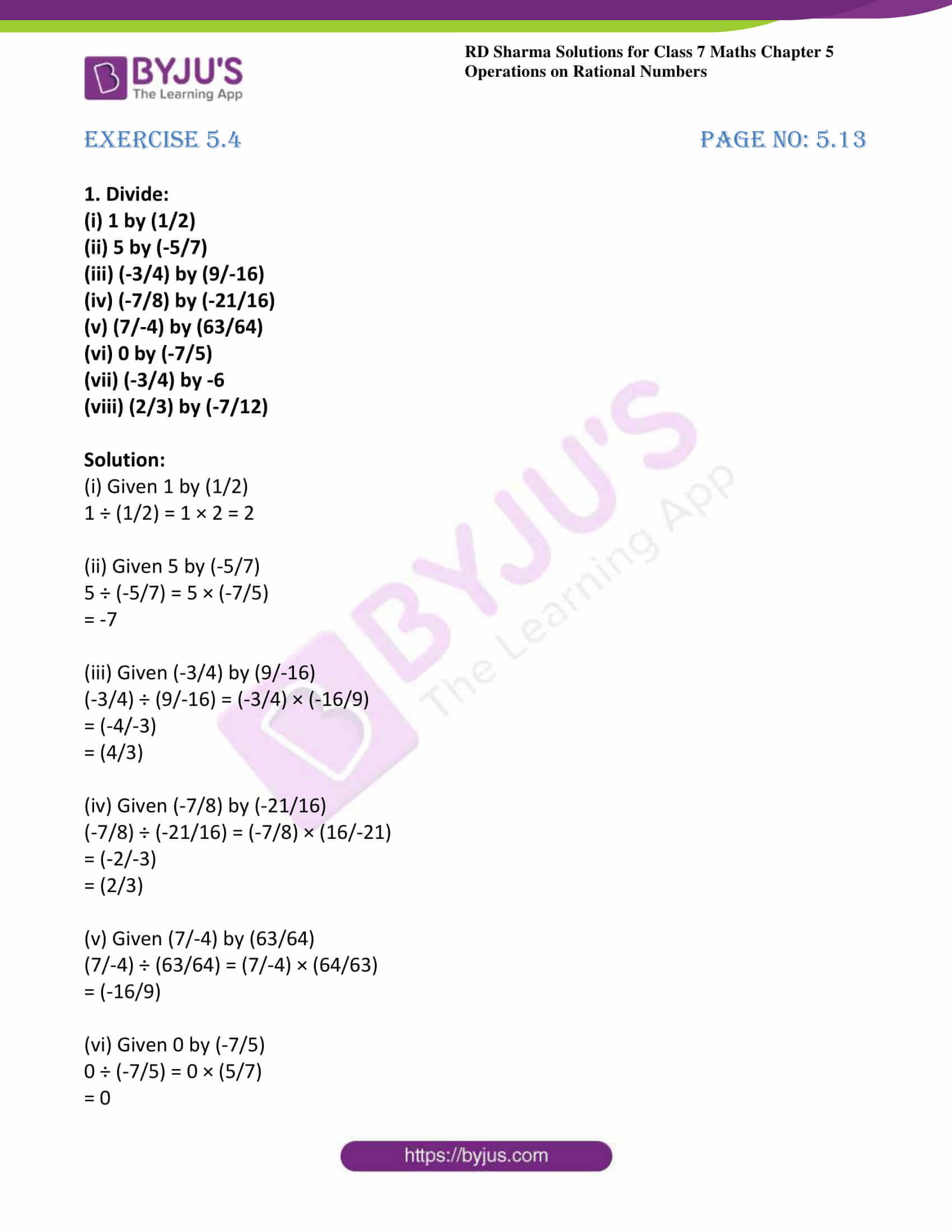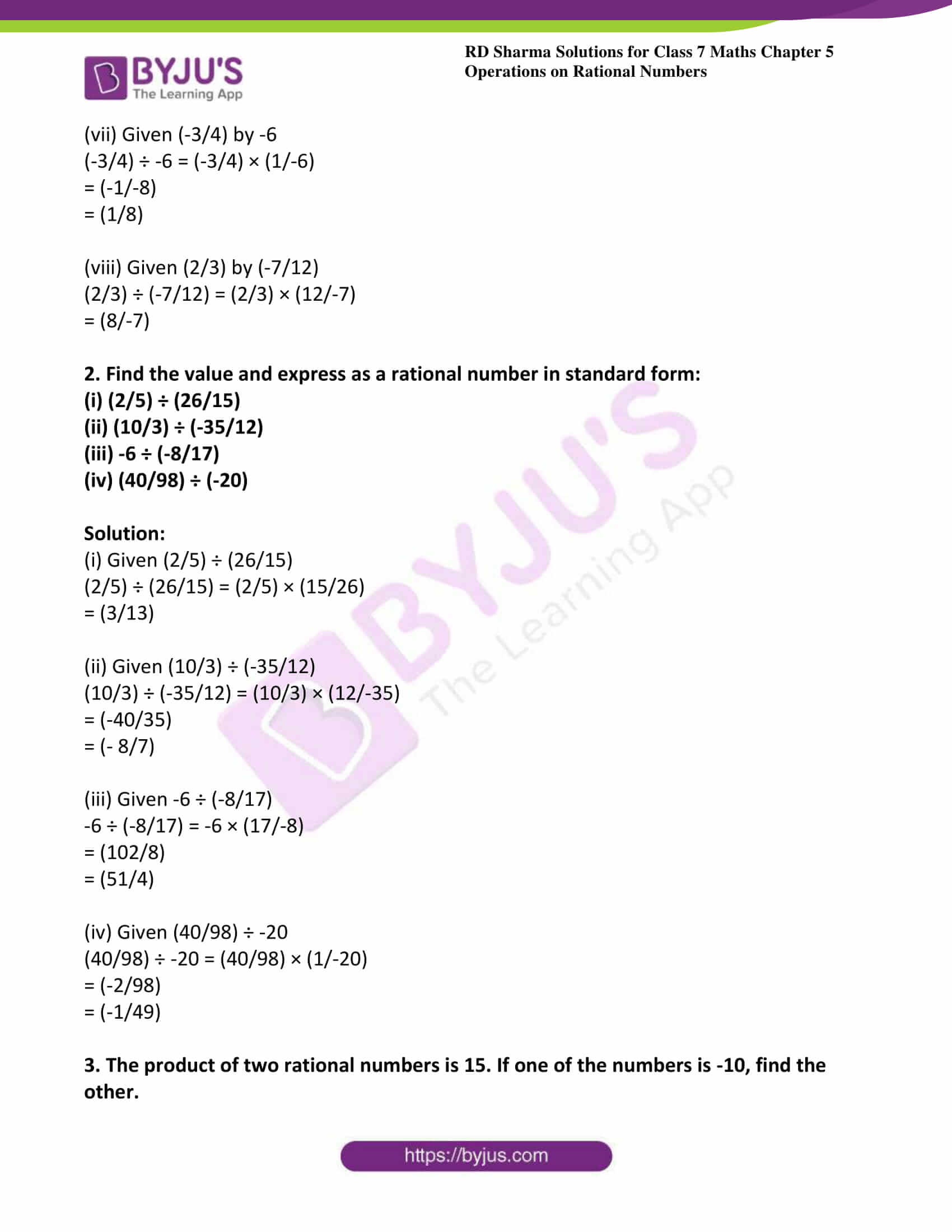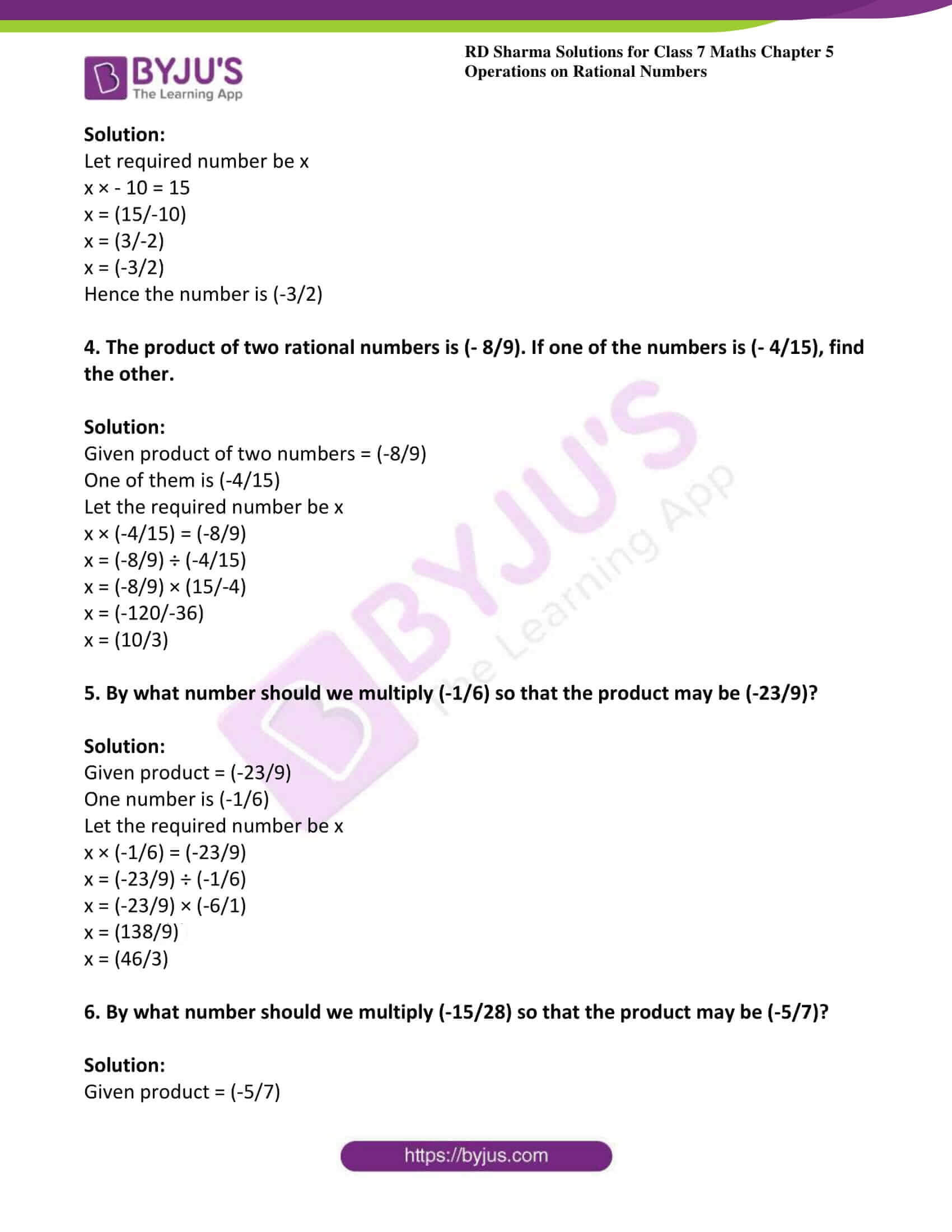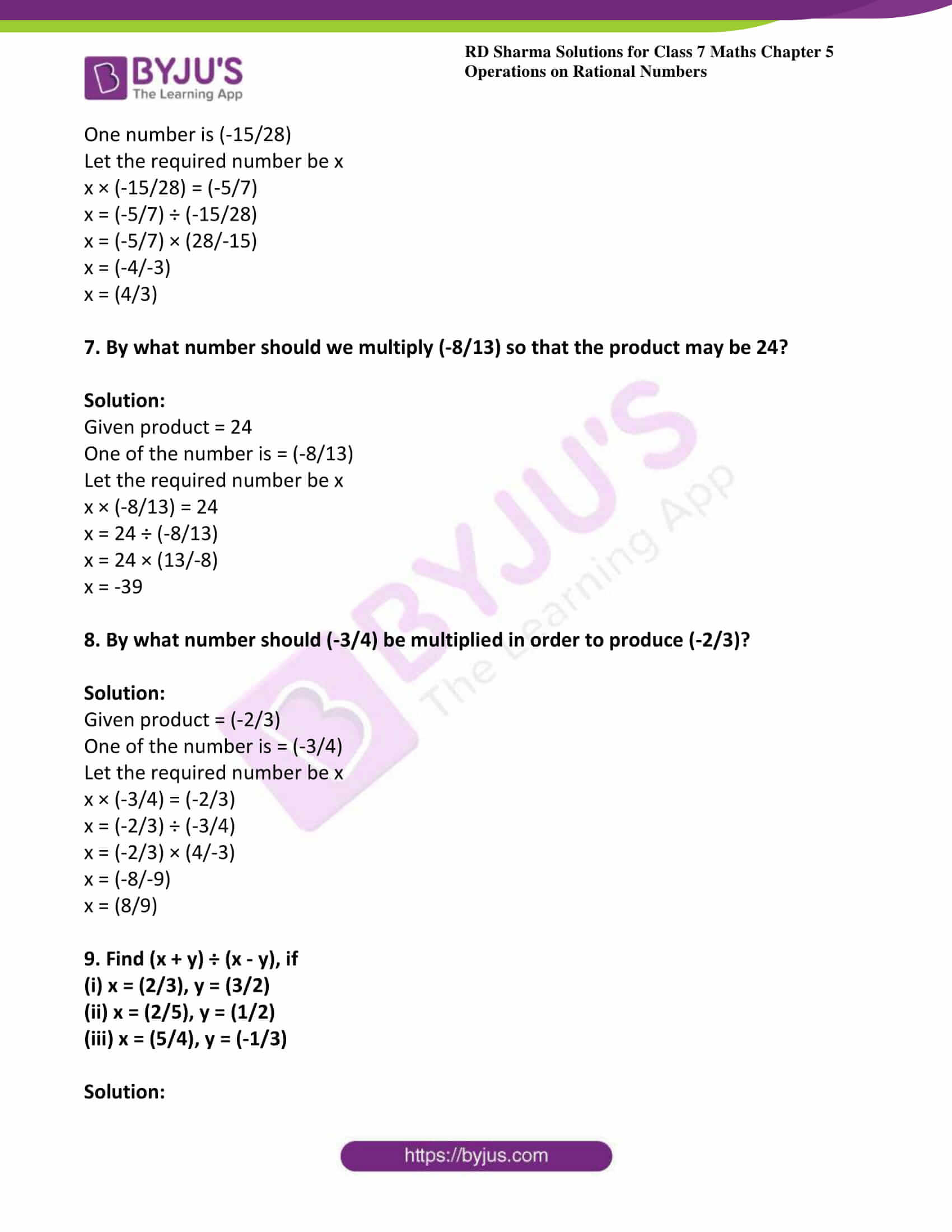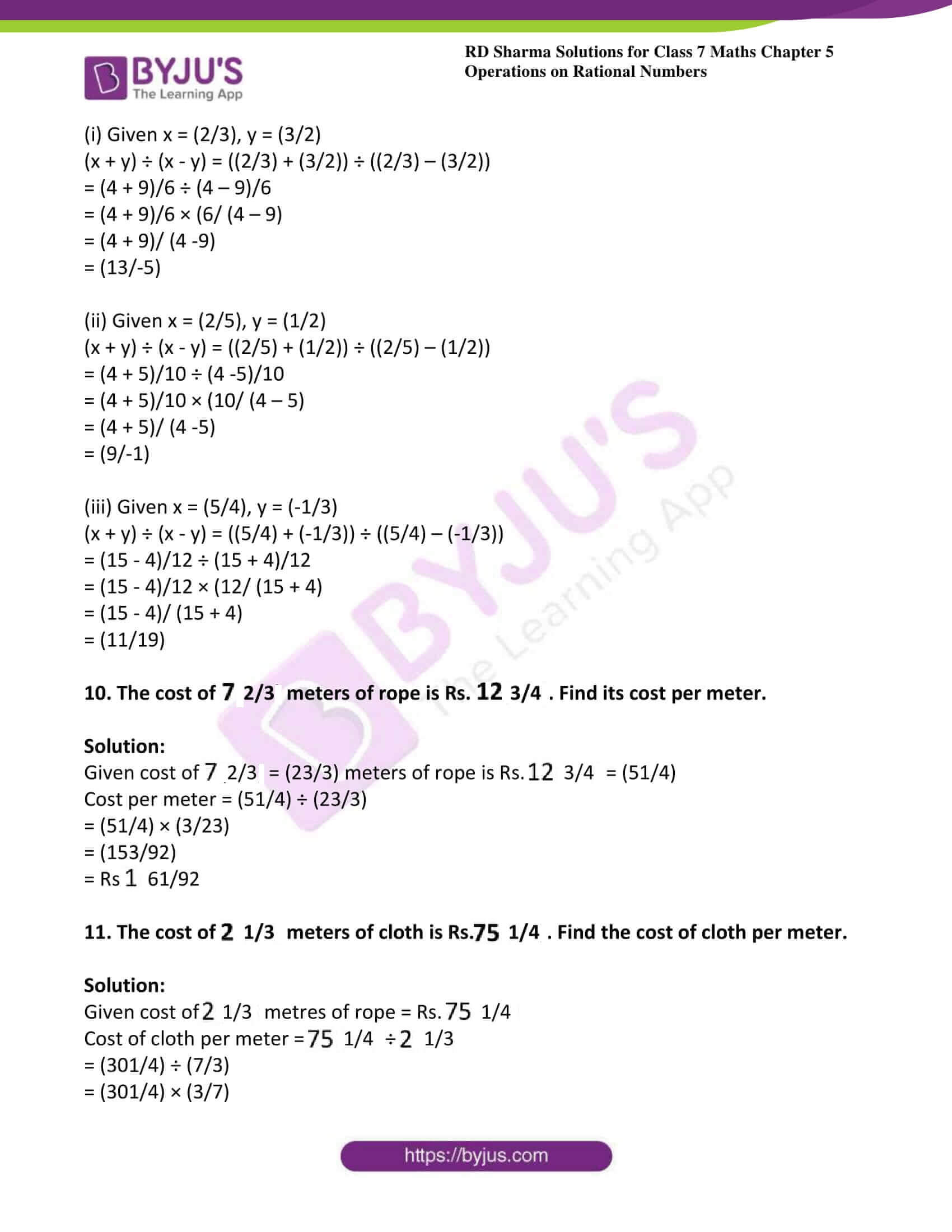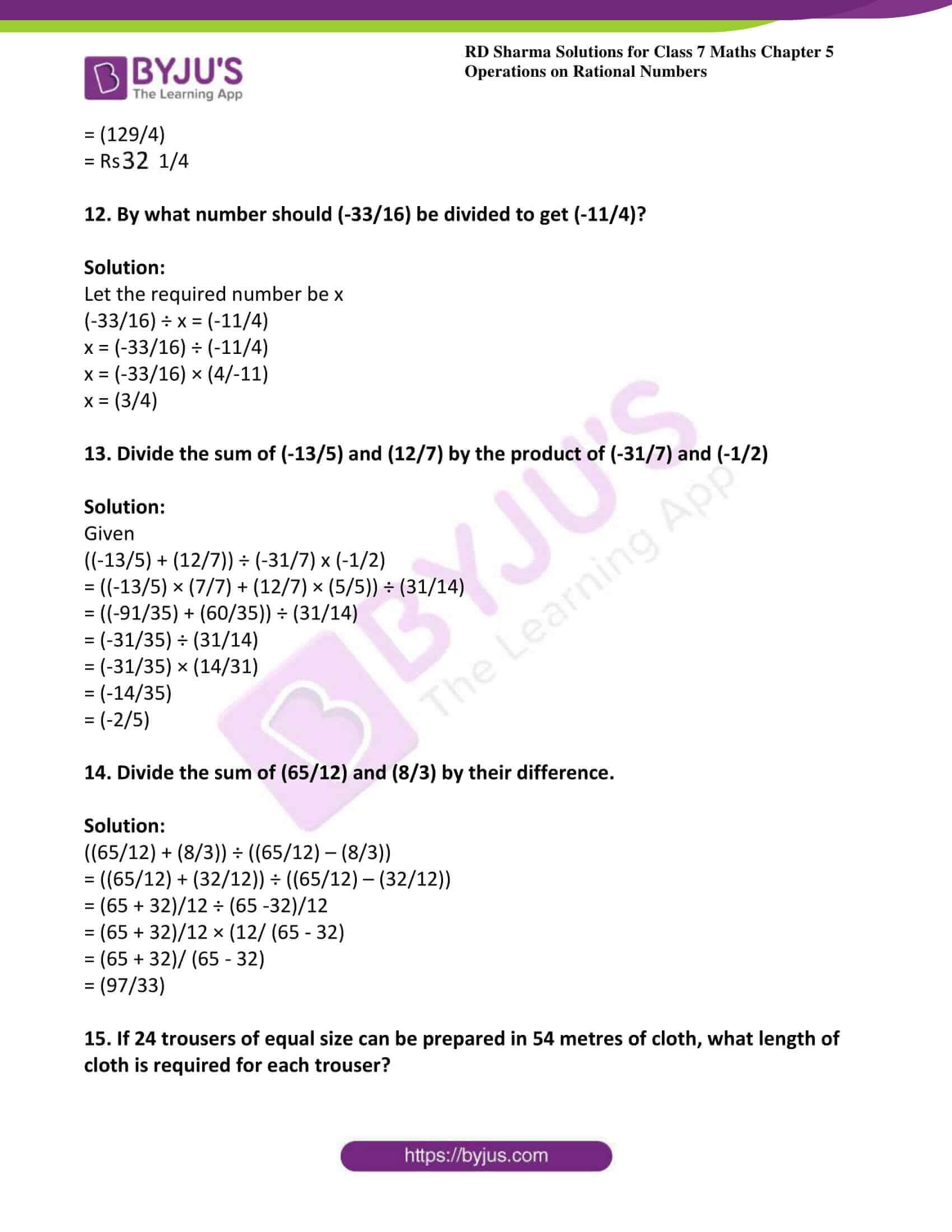### Access answers to Maths RD Sharma Solutions For Class 7 Chapter 5 – Operations On Rational Numbers Exercise 5.4

1. Divide:

(i) 1 by (1/2)

(ii) 5 by (-5/7)

(iii) (-3/4) by (9/-16)

(iv) (-7/8) by (-21/16)

(v) (7/-4) by (63/64)

(vi) 0 by (-7/5)

(vii) (-3/4) by -6

(viii) (2/3) by (-7/12)

Solution:

(i) Given 1 by (1/2)

1 ÷ (1/2) = 1 × 2 = 2

(ii) Given 5 by (-5/7)

5 ÷ (-5/7) = 5 × (-7/5)

= -7

(iii) Given (-3/4) by (9/-16)

(-3/4) ÷ (9/-16) = (-3/4) × (-16/9)

= (-4/-3)

= (4/3)

(iv) Given (-7/8) by (-21/16)

(-7/8) ÷ (-21/16) = (-7/8) × (16/-21)

= (-2/-3)

= (2/3)

(v) Given (7/-4) by (63/64)

(7/-4) ÷ (63/64) = (7/-4) × (64/63)

= (-16/9)

(vi) Given 0 by (-7/5)

0 ÷ (-7/5) = 0 × (5/7)

= 0

(vii) Given (-3/4) by -6

(-3/4) ÷ -6 = (-3/4) × (1/-6)

= (-1/-8)

= (1/8)

(viii) Given (2/3) by (-7/12)

(2/3) ÷ (-7/12) = (2/3) × (12/-7)

= (8/-7)

2. Find the value and express as a rational number in standard form:

(i) (2/5) ÷ (26/15)

(ii) (10/3) ÷ (-35/12)

(iii) -6 ÷ (-8/17)

(iv) (40/98) ÷ (-20)

Solution:

(i) Given (2/5) ÷ (26/15)

(2/5) ÷ (26/15) = (2/5) × (15/26)

= (3/13)

(ii) Given (10/3) ÷ (-35/12)

(10/3) ÷ (-35/12) = (10/3) × (12/-35)

= (-40/35)

= (- 8/7)

(iii) Given -6 ÷ (-8/17)

-6 ÷ (-8/17) = -6 × (17/-8)

= (102/8)

= (51/4)

(iv) Given (40/98) ÷ -20

(40/98) ÷ -20 = (40/98) × (1/-20)

= (-2/98)

= (-1/49)

3. The product of two rational numbers is 15. If one of the numbers is -10, find the other.

Solution:

Let required number be x

x × – 10 = 15

x = (15/-10)

x = (3/-2)

x = (-3/2)

Hence the number is (-3/2)

4. The product of two rational numbers is (- 8/9). If one of the numbers is (- 4/15), find the other.

Solution:

Given product of two numbers = (-8/9)

One of them is (-4/15)

Let the required number be x

x × (-4/15) = (-8/9)

x = (-8/9) ÷ (-4/15)

x = (-8/9) × (15/-4)

x = (-120/-36)

x = (10/3)

5. By what number should we multiply (-1/6) so that the product may be (-23/9)?

Solution:

Given product = (-23/9)

One number is (-1/6)

Let the required number be x

x × (-1/6) = (-23/9)

x = (-23/9) ÷ (-1/6)

x = (-23/9) × (-6/1)

x = (138/9)

x = (46/3)

6. By what number should we multiply (-15/28) so that the product may be (-5/7)?

Solution:

Given product = (-5/7)

One number is (-15/28)

Let the required number be x

x × (-15/28) = (-5/7)

x = (-5/7) ÷ (-15/28)

x = (-5/7) × (28/-15)

x = (-4/-3)

x = (4/3)

7. By what number should we multiply (-8/13) so that the product may be 24?

Solution:

Given product = 24

One of the number is = (-8/13)

Let the required number be x

x × (-8/13) = 24

x = 24 ÷ (-8/13)

x = 24 × (13/-8)

x = -39

8. By what number should (-3/4) be multiplied in order to produce (-2/3)?

Solution:

Given product = (-2/3)

One of the number is = (-3/4)

Let the required number be x

x × (-3/4) = (-2/3)

x = (-2/3) ÷ (-3/4)

x = (-2/3) × (4/-3)

x = (-8/-9)

x = (8/9)

9. Find (x + y) ÷ (x – y), if

(i) x = (2/3), y = (3/2)

(ii) x = (2/5), y = (1/2)

(iii) x = (5/4), y = (-1/3)

Solution:

(i) Given x = (2/3), y = (3/2)

(x + y) ÷ (x – y) = ((2/3) + (3/2)) ÷ ((2/3) – (3/2))

= (4 + 9)/6 ÷ (4 – 9)/6

= (4 + 9)/6 × (6/ (4 – 9)

= (4 + 9)/ (4 -9)

= (13/-5)

(ii) Given x = (2/5), y = (1/2)

(x + y) ÷ (x – y) = ((2/5) + (1/2)) ÷ ((2/5) – (1/2))

= (4 + 5)/10 ÷ (4 -5)/10

= (4 + 5)/10 × (10/ (4 – 5)

= (4 + 5)/ (4 -5)

= (9/-1)

(iii) Given x = (5/4), y = (-1/3)

(x + y) ÷ (x – y) = ((5/4) + (-1/3)) ÷ ((5/4) – (-1/3))

= (15 – 4)/12 ÷ (15 + 4)/12

= (15 – 4)/12 × (12/ (15 + 4)

= (15 – 4)/ (15 + 4)

= (11/19)

10. The cost of $$7\frac{2}{3}$$ meters of rope is Rs. $$12\frac{3}{4}$$. Find its cost per meter.

Solution:

Given cost of $$7\frac{2}{3}$$ = (23/3) meters of rope is Rs. $$12\frac{3}{4}$$ = (51/4)

Cost per meter = (51/4) ÷ (23/3)

= (51/4) × (3/23)

= (153/92)

= Rs $$1\frac{61}{92}$$

11. The cost of $$2\frac{1}{3}$$ meters of cloth is Rs.$$75\frac{1}{4}$$. Find the cost of cloth per meter.

Solution:

Given cost of $$2\frac{1}{3}$$ metres of rope = Rs. $$75\frac{1}{4}$$

Cost of cloth per meter = $$75\frac{1}{4}$$ ÷ $$2\frac{1}{3}$$

= (301/4) ÷ (7/3)

= (301/4) × (3/7)

= (129/4)

= Rs $$32\frac{1}{4}$$

12. By what number should (-33/16) be divided to get (-11/4)?

Solution:

Let the required number be x

(-33/16) ÷ x = (-11/4)

x = (-33/16) ÷ (-11/4)

x = (-33/16) × (4/-11)

x = (3/4)

13. Divide the sum of (-13/5) and (12/7) by the product of (-31/7) and (-1/2)

Solution:

Given

((-13/5) + (12/7)) ÷ (-31/7) x (-1/2)

= ((-13/5) × (7/7) + (12/7) × (5/5)) ÷ (31/14)

= ((-91/35) + (60/35)) ÷ (31/14)

= (-31/35) ÷ (31/14)

= (-31/35) × (14/31)

= (-14/35)

= (-2/5)

14. Divide the sum of (65/12) and (8/3) by their difference.

Solution:

((65/12) + (8/3)) ÷ ((65/12) – (8/3))

= ((65/12) + (32/12)) ÷ ((65/12) – (32/12))

= (65 + 32)/12 ÷ (65 -32)/12

= (65 + 32)/12 × (12/ (65 – 32)

= (65 + 32)/ (65 – 32)

= (97/33)

15. If 24 trousers of equal size can be prepared in 54 metres of cloth, what length of cloth is required for each trouser?

Solution:

Given material required for 24 trousers = 54m

Cloth required for 1 trouser = (54/24)

= (9/4) meters